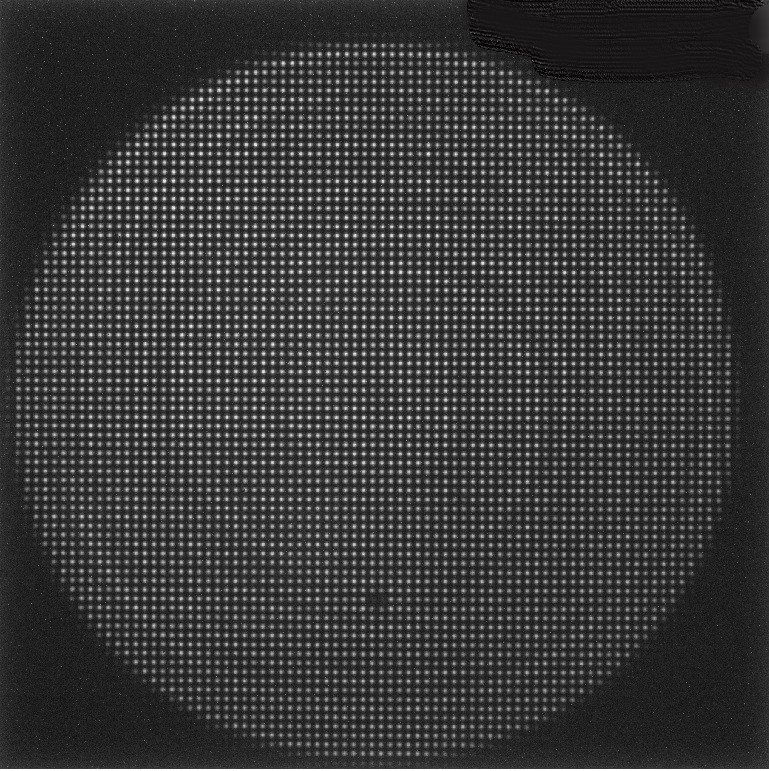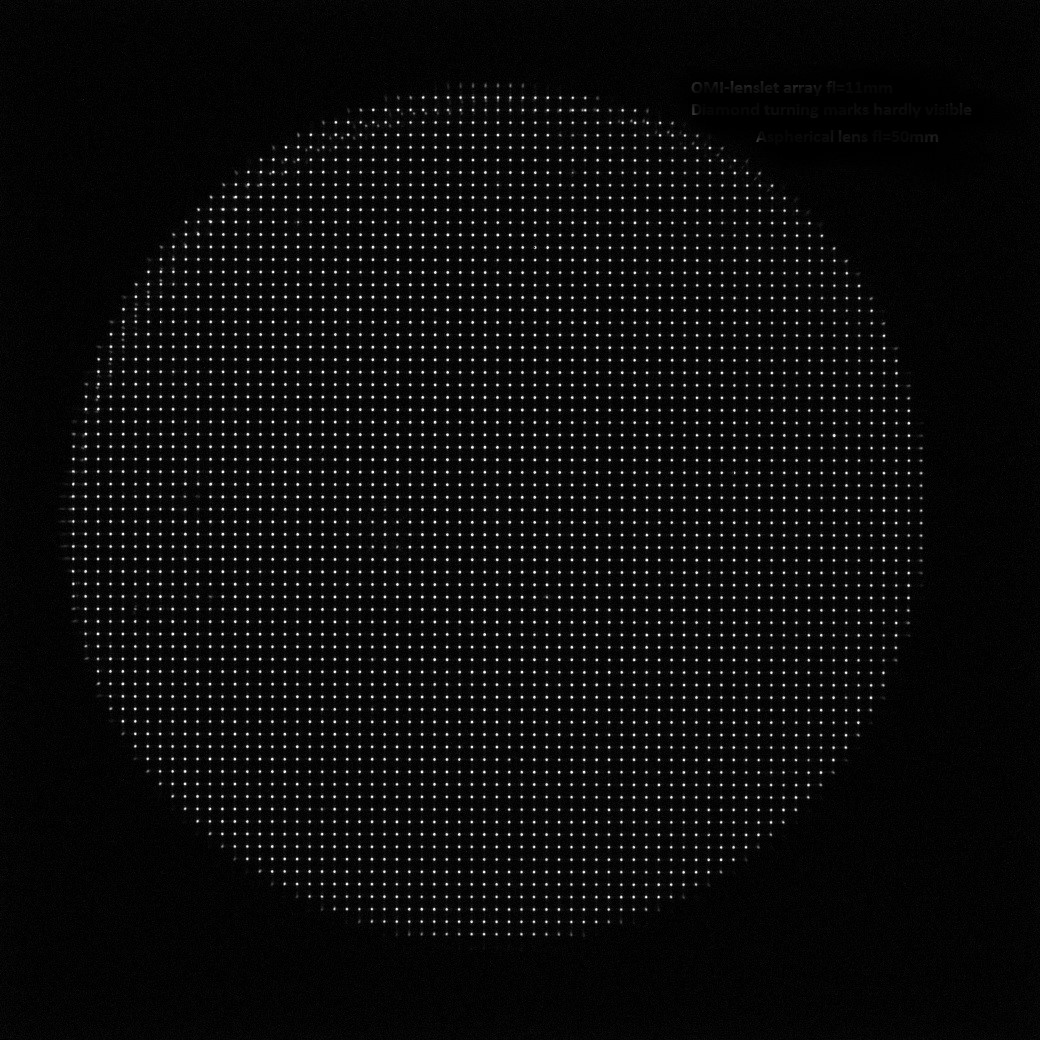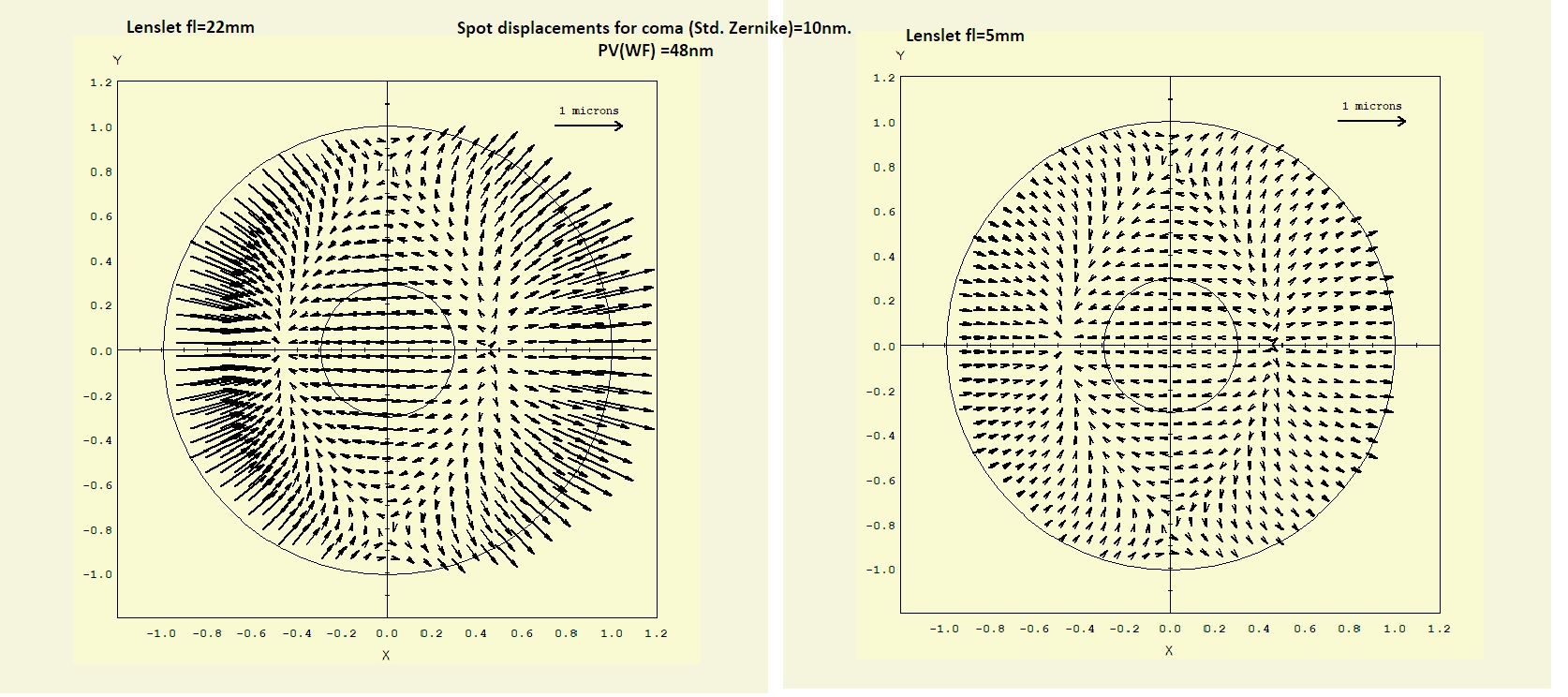# Importance of using lenslet arrays with long focal length

#### Shack-Hartmann image of aspherical lens fl=50mm taken with OMI lenslet array fl=22mm and fl=11mmShack-Hartmann image taken with OMI-22 (lenslet array with fl=22mm). The diamond turning marks are clearly visibleShack-Hartmann image taken with OMI-11 (lenslet array with fl=11mm). The diamond turning marks are hardly visible

We explain the importance of using Shack-Hartmann lenslet array with long focal length using a simulation computed with our Sensoft simulation package.

10 nm of Coma are added to a perfect wave (Standard Zernike coefficients). The spot displacements on the normalized pupil are computed for two different cases:

• assuming our standard lenslet array with focal length = 22 mm
• assuming a generic array with focal length = 5 mm

The result is in Figure below. Since the length of the displacement is proportional to the focal length it is quite evident that it will be easier to detect Coma when fl= 22 mm

Even more interesting is the next simulation when we add 0.2 micron random noise to the displacements again for the 2 cases above. Results are in figure 2: in presence of modest noise, with fl=5 mm the Coma displacements disappear inside the noise while for fl=22 mm  the Coma pattern is still visible in the displacements

Figure 1. Spot displacements due to Coma aberration (PV = 48 nm) that one can expect using Shack-Hartmann lenslet array with focal length fl= 22 mm  (our standard choice, left) and  fl=5 mm (right)Figure 2. Adding random of max 0.2 um. For lenslet array with focal length fl= 5 mm (right) the displacements are inside the noise while for fl=22 mm (left) the Coma pattern is still visible in the displacements### Conclusions:

• Longer focal length means higher accuracy: deviation on camera chip is proportional to focal length
• Longer focal length means coarser sampling.  Because of that, we improve the sampling by a factor ~3X using sophisticated mathematical interpolation
• Short focal length gives unstable and unreliable results since it is very poor in resolving aberrations especially in presence of noise and also causes thermal instability as the lenslet array is too close to the camera chip and INSIDE the camera body